# OHM'S LAW

Click or Tap the Example circuits below to invoke TINACloud and select the Interactive DC mode to Analyze them Online.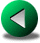THREE PHASE NETWORKSSERIES CONNECTED RESISTORS

The magnitude of current flowing in a circuit is dependent upon both voltage and resistance. The relationship among these three electrical properties (current, voltage and resistance) is the important and well-known Ohm's law, which states that the current flow in a circuit is directly proportional to the applied source voltage and inversely proportional to the resistance of the circuit.

In mathematical form: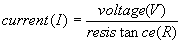or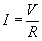In the following examples, we generally give three solutions for each problem.

• Numerical solution by TINA
• Solution by TINA's Interpreter, using Ohm's law
• Solution by formulas using Ohm's law

#### Example 1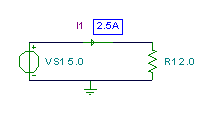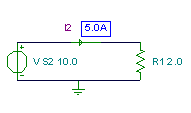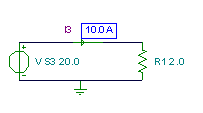Click/tap the circuit above to analyze on-line or click this link to Save under Windows

Using Ohm's law: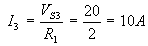The calculated currents confirm that current is directly proportional to the source voltage.

{Using TINA's Interpreter}
I1:=VS1/R1;
I1=[2.5]
I2:=VS2/R1;
I2=
I3:=VS3/R1;
I3=

#### Example 2

In the following example, you can verify that the current is inversely proportional to the resistance.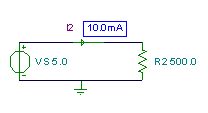Click/tap the circuit above to analyze on-line or click this link to Save under Windows

Using Ohm's law: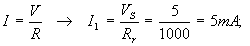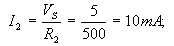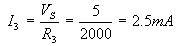{Using TINA's Interpreter}
I1:=VS/R1;
I1=[5m]
I2:=VS/R2;
I2=[10m]
I3:=VS/R3;
I3=[2.5m]

#### Example 3

In this example, you can see that the voltage across a resistor is directly proportional to the value of its resistance.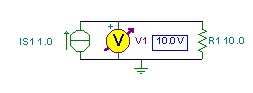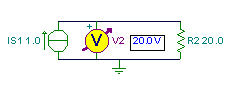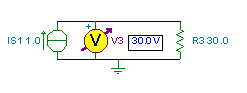Click/tap the circuit above to analyze on-line or click this link to Save under Windows

While the detailed formula isn't shown, it is used in TINA's Interpreter to evaluate the example.
{Using TINA's Interpreter}
V1:=IS1*R1;
V1=
V2:=IS1*R2;
V2=
V3:=IS1*R3;
V3=

#### Example 4

In this example, you can check that the voltage across a resistor is directly proportional to the current flowing through the resistor and to the resistor's resistance.

Click/tap the circuit above to analyze on-line or click this link to Save under Windows

While the detailed formula isn't shown, it is used in TINA's Interpreter to evaluate the example.
{Using TINA's Interpreter}
V1:=IS1*R1;
V1=
V2:=IS2*R1;
V2=
V3:=IS3*R1;
V3=THREE PHASE NETWORKSSERIES CONNECTED RESISTORS